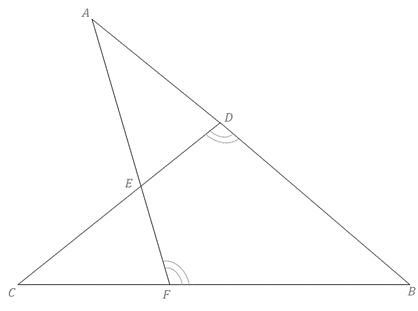Chapter 5.3, Problem 32EElementary Geometry For College St...

7th Edition
Alexander + 2 others
ISBN: 9781337614085

Solutions

Chapter
SectionElementary Geometry For College St...

7th Edition
Alexander + 2 others
ISBN: 9781337614085
Textbook Problem

Δ A B F ∼ Δ C B D with obtuse angles at vertices D and F as indicated. If m ∠ B = 44 ° , and m ∠ A : m ∠ C D B = 1 : 3 , find m ∠ A .Exercises 31, 32

To determine

To find:

The degree of A by using the given information.

Explanation

Definition:

CASTC:

Corresponding angles of similar triangles are congruent.

Description:

Given that ΔABFΔCBD with obtuse angles at vertices D and F as indicated. If mB=44°, and mA:mCDB=1:3. That is, mAmCDB=x3x.

Hence, mA=x and mCDB=3x.

The given figure is shown below.

Figure 1

From the given figure, it is observed that there are two triangles ABF and CBD and which is given that two triangles are similar.

Now draw the two triangles separately as shown below.

Still sussing out bartleby?

Check out a sample textbook solution.

See a sample solution

The Solution to Your Study Problems

Bartleby provides explanations to thousands of textbook problems written by our experts, many with advanced degrees!

Get Started

Evaluate the expression sin Exercises 116. 2322

Finite Mathematics and Applied Calculus (MindTap Course List)

In Exercises 35-42, find functions f and g such that h = g f. (Note: The answer is not unique.) 40. h(x)=1x24

Applied Calculus for the Managerial, Life, and Social Sciences: A Brief Approach

0 1 −∞ does not exist

Study Guide for Stewart's Single Variable Calculus: Early Transcendentals, 8th